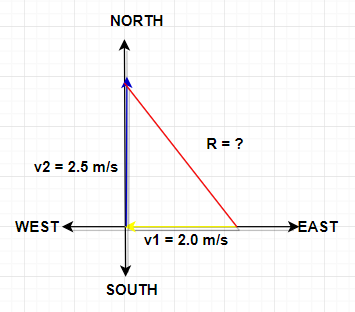# A ferry is crossing a river.The ferry is headed due north with a speed of 2.5 m/s relative to the...

## Question:

A ferry is crossing a river. The ferry is headed due north with a speed of 2.5 m/s relative to the water and the river's velocity is 2.0 m/s to the east. What is the magnitude and direction of the ferry's velocity relative to Earth? ( Give the angle from east 0 degrees )

Using head-to-tail method in this problem we were able to obtain a triangle, because of this we can use the Pythagorean theorem. This theorem states that the square of the length of the hypotenuse is equivalent to the squares of the sum of the length of the two sides.Given :

• River's water velocity (East) : {eq}v_1 = 2.0\ \rm m/s {/eq}
• Ferry's velocity(North) : {eq}v_2 = 2.5\ \rm m/s{/eq}

Find :

• magnitude and direction of the ferry's velocity : {eq}v_r = ?{/eq}

Using the head-to-tail method the vectors in our figure produce a triangle shape with a resultant vector.

With this, we can now solve the velocity of the plane using the Pythagorean theorem or vector addition.

\begin{align} R^2 &= A^2+B^2 \\ v_r^2 &= v_1^2+v_2^2 \\ v_r & = \sqrt {(2.0\ \rm m/s)^2+(2.5\ \rm m/s)^2}\\ v_r& = \sqrt {10.25\ \rm m^2/s^2}\\ v_r & = \boxed{3.2\ \rm m/s} \end{align}

To solve for the direction we will use the formula,

\begin{align} \theta &= \tan^{-1}\left(\frac{\sum y}{\sum x} \right) \\ \theta &= \tan^{-1}\left(\frac{2.5\ \rm m/s}{2.0\ \rm m/s } \right) \\ \theta &\approx \boxed{51.3^{\circ}} \end{align}